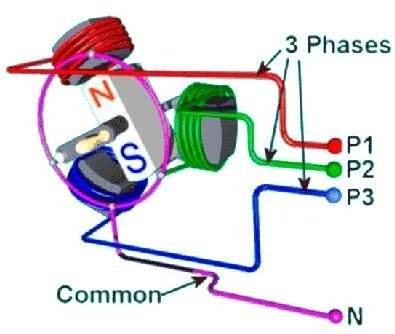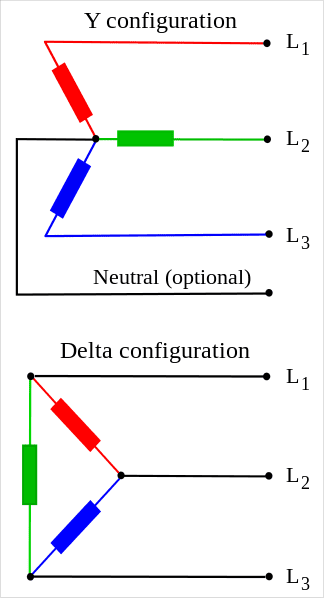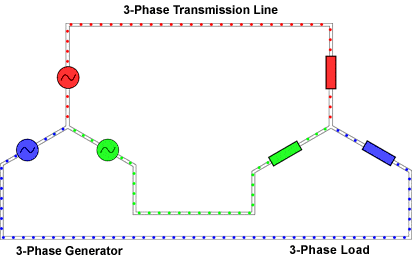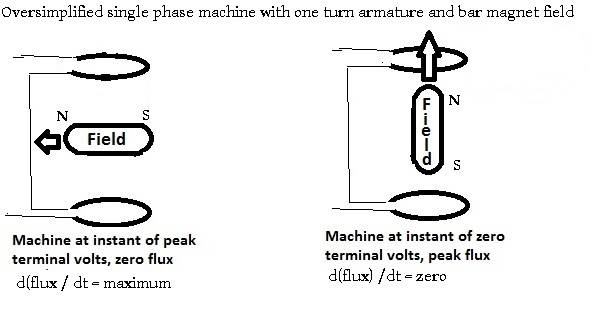# Fundamental confusion about THREE-PHASE electric power setup

• s3a
In summary, the three lines in a Wye/Y configuration or Delta/Δ configuration can be connected to produce three power sources that are 120 degrees out of phase with each other. The generator is started by providing external mechanical input. The sole purpose of a generator is to convert mechanical energy into electrical energy.

## Homework Statement

Hello to everyone who's reading. :)

I hope I posted this in the right place; while this is not exactly a homework problem, I am trying to improve my understanding of the background information before proceeding with course-style problems.

This post is because I'm trying to fully understand the fundamentals of the topic of three-phase electric power setups, but I feel like I don't fully get it, despite searching online for several days.

## Homework Equations

Three-phaseElectricPower.jpeg image:WyeDelta.png image:3-phase_flow.gif image:## The Attempt at a Solution

Basically, here is what I believe I do understand.:
1. The three lines can be connected in a Wye / Y configuration or in a Delta / Δ configuration, like shown in the WyeDelta.png image.: (There can optionally be a fourth line that's neutral in a Wye / Y configuration.)
2. Somehow, there are three power sources, and those power sources are are plus or minus 120 degrees out of phase from each of the other two power sources. (Here, I use the word "somehow" to emphasize my third confusion (shown below - not the #3 directly below, but the one in the next section).)
3. A change in magnetic field induces current (by producing an electromotive force across the conductor in question).
4. Magnetic fields are produced by moving charges, which includes electric current.
Here are things I'm struggling with.:
1. Are the three coils that surround the magnet (for example, as in the image Three-phaseElectricPower.jpeg) or are surrounded by the two magnets inductors (as I've seen in another source)?
2. If the coils that surround the magnet or are surrounded by the two magnets are in fact inductors, then are their impedances just ignored because of it not mattering due to voltage sources delivering the "promised" voltage anyways? In other words, do the impedances in the 3-phase_flow.gif image only represent the impedance that is not present at the site of the electromagnetic induction / magnetic induction?
3. How does the generator start? Do the three power sources start the rotation of the magnet or does the rotation of the magnet start the power providing of the power sources? Or, what else?
Any input that could help me improve my understanding would be greatly appreciated!

#### Attachments

•Klystron and nrqed
The generator in your diagram is a just a model for understanding three-phase generation. Practical generators are constructed differently.

s3a said:
Are the three coils that surround the magnet (for example, as in the image Three-phaseElectricPower.jpeg) or are surrounded by the two magnets inductors (as I've seen in another source)?
The rotating bar magnet is surrounded by the three coils (electromagnets).
s3a said:
If the coils that surround the magnet or are surrounded by the two magnets are in fact inductors, then are their impedances just ignored because of it not mattering due to voltage sources delivering the "promised" voltage anyways? In other words, do the impedances in the 3-phase_flow.gif image only represent the impedance that is not present at the site of the electromagnetic induction / magnetic induction?
The flux from the rotating bar magnet which links with the coils produces emf. The current depends on the load impedance, the leakage reactance of the coils and the synchronous reactance of the machine (assuming zero resistance in the conductors). Synchronous reactance is a bit difficult to explain. We can get back to that later if you want.
s3a said:
How does the generator start? Do the three power sources start the rotation of the magnet or does the rotation of the magnet start the power providing of the power sources? Or, what else?
Any generator is started and run by providing external mechanical input. The sole purpose of a generator is to convert mechanical energy into electrical energy.
In power stations, this mechanical input (torque) is derived from coal, hydro, nuclear energies.

•Klystron and jim hardy
Interesting diagram. Consider plotting the output of phase 1 over time assuming the 3-phase generator running at steady RPM. Then plot phase-2 output at the same t0 under phase-1; then phase-3. Drawing a pencil and graph-paper plot with each phase vertically stacked illustrates the relationships among the 3-phase output over time. Actual values are not required to visualize the phase-shifts among the 3 outputs.

Superimpose the plots -- admittedly easier using plotting software -- to more thoroughly understand how each phase varies as the rotating magnet impinges on each coil. Plotting a vertical line normal to the x-axis through each phase illustrates 'what the load sees' from the combined outputs.

A beginning engineer might plot output Voltage x time, then Current x time for each phase and analyze and compare the sine wave graphs to further understand Impedance. From here, plotting induced EMF over time helps understand the power generated by the rotating flux.

Consider efficiency. Why use 3-phases? Why complicate the generator by adding coils to the stator? How does using the Y configuration differ from Delta config?

This USGS hydro-electric article illustrates how energy of falling water converts to EMF.
https://water.usgs.gov/edu/hyhowworks.html

[I haven't taught recently. Please edit my errors or clarify the concepts. --Norm]

Last edited:
•jim hardy
Are you familiar with phasor notation from elementary AC circuit analysis ?

It's simply that you have three rotating phasors not just one.

If you wanted to you could mount three identical single phase generators on one shaft, offset them from one another by 1/3 turn, and have a really ugly but easy to understand three phase generator.

To get you started here's the simplest mental model for an alternator.
It's just a bar magnet rotating between two coils.
The coils are called the armature.
Since flux leaves one end of the bar magnet and returns to the other, you might as well use a coil at each end of it and generate twice the voltage. (Why waste good flux ?)
They're just coils and coils have inductance. So to your question above i'd call them inductors not electromagnets.
(caveat - definition of inductance is flux linkages per ampere, so every inductor can be an electromagnet if you let current flow in it. But that's for later on, i mention it here only for honesty's sake- jh)
As the bar magnet rotates, its magnetic flux going through the armature coils alternates direction passing through zero midway between peaks.
That changing flux induces voltage in the armature coils.Now recall Faraday tells us e = dΦ/dt , so there's a derivative-integral relation between flux and voltage.
Also recall that for a sine wave, which AC power almost universally is, differentiation shifts phase ninety degrees. Trig identities d(sin) = cosine et al ?
That's why the quarter turn between peak flux and peak armature voltage.

If you mount three of those on one shaft you'll have a three phase machine.

In a generator the magnet is spun by some external machine - hand crank, gas engine, steam turbine for example.
To make a motor one instead connects three phase power to the armature.
Armature current flows and makes a rotating magnetic field that drags the bar magnet around.
In that case you could call the coils "electromagnets", but we just call them "armature".
Difference between a motor and generator is only the direction of power flow.
Same machine can do both jobs. When it's a motor it delivers torque, when a generator it absorbs torque.

.......................

s3a said:
Are the three coils that surround the magnet (for example, as in the image Three-phaseElectricPower.jpeg) or are surrounded by the two magnets inductors (as I've seen in another source)?
Just call them 'armature coils' and remember the definition of inductance. Any coil becomes an electromagnet when current flows in it.

s3a said:
If the coils that surround the magnet or are surrounded by the two magnets are in fact inductors, then are their impedances just ignored because of it not mattering due to voltage sources delivering the "promised" voltage anyways? In other words, do the impedances in the 3-phase_flow.gif image only represent the impedance that is not present at the site of the electromagnetic induction / magnetic induction?
Think about that for a minute.
An ideal voltage source has zero impedance.
When you make a Thevenin's equivalent circuit for a generator you will need to find the 'impedance' of its armature coils.
It had best be small compared to that of the load else you have a really inefficient generator.

s3a said:
How does the generator start? Do the three power sources start the rotation of the magnet or does the rotation of the magnet start the power providing of the power sources? Or, what else?

..........................

The way i was taught three phase was as three individual single phase voltage phasors joined at the wye.
Label the phases A B C and the junction N.
Then draw your phasors . Be rigorous in naming them from-to, ie Vna Vnb Vnc .
...
Then you'll see that Vab - Vnb + Vna = 0
Excruciating Rigor in naming and drawing the phasors is vital to success with 3phase.

I hope the above helps. I'm no teacher , just had a good one fifty years ago.

old jim

#### Attachments

Last edited:
•Klystron, cnh1995 and anorlunda